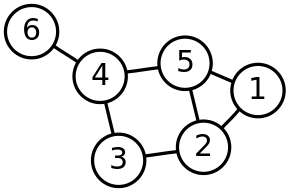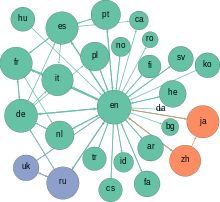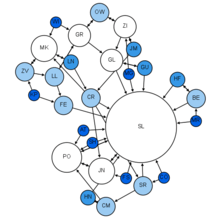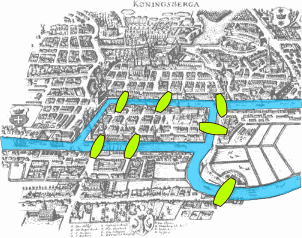# Graph theoryA drawing of a graph

In mathematics graph theory is the study of graphs, which are mathematical structures used to model pairwise relations between objects. A graph in this context is made up of vertices, nodes, or points which are connected by edges, arcs, or lines. A graph may be undirected, meaning that there is no distinction between the two vertices associated with each edge, or its edges may be directed from one vertex to another; see Graph (discrete mathematics) for more detailed definitions and for other variations in the types of graph that are commonly considered. Graphs are one of the prime objects of study in discrete mathematics.

Refer to the glossary of graph theory for basic definitions in graph theory.

## Definitions

Definitions in graph theory vary. The following are some of the more basic ways of defining graphs and related mathematical structures.

### Graph

In the most common sense of the term, a graph is an ordered pair G = (V, E) comprising a set V of vertices or nodes or points together with a set E of edges or arcs or lines, which are 2-element subsets of V (i.e. an edge is associated with two vertices, and that association takes the form of the unordered pair comprising those two vertices). To avoid ambiguity, this type of graph may be described precisely as undirected and simple.

Other senses of graph stem from different conceptions of the edge set. In one more generalized notion, V is a set together with a relation of incidence that associates with each edge two vertices. In another generalized notion, E is a multiset of unordered pairs of (not necessarily distinct) vertices. Many authors call this type of object a multigraph or pseudograph.

All of these variants and others are described more fully below.

The vertices belonging to an edge are called the ends or end vertices of the edge. A vertex may exist in a graph and not belong to an edge.

V and E are usually taken to be finite, and many of the well-known results are not true (or are rather different) for infinite graphs because many of the arguments fail in the infinite case. The order of a graph is |V|, its number of vertices. The size of a graph is |E|, its number of edges. The degree or valency of a vertex is the number of edges that connect to it, where an edge that connects a vertex to itself (a loop) is counted twice.

For an edge {x, y}, graph theorists usually use the somewhat shorter notation xy.

## ApplicationsThe network graph formed by Wikipedia editors (edges) contributing to different Wikipedia language versions (vertices) during one month in summer 2013

Graphs can be used to model many types of relations and processes in physical, biological, social and information systems. Many practical problems can be represented by graphs. Emphasizing their application to real-world systems, the term network is sometimes defined to mean a graph in which attributes (e.g. names) are associated with the nodes and/or edges.

In computer science, graphs are used to represent networks of communication, data organization, computational devices, the flow of computation, etc. For instance, the link structure of a website can be represented by a directed graph, in which the vertices represent web pages and directed edges represent links from one page to another. A similar approach can be taken to problems in social media, travel, biology, computer chip design, and many other fields. The development of algorithms to handle graphs is therefore of major interest in computer science. The transformation of graphs is often formalized and represented by graph rewrite systems. Complementary to graph transformation systems focusing on rule-based in-memory manipulation of graphs are graph databases geared towards transaction-safe, persistent storing and querying of graph-structured data.

Graph-theoretic methods, in various forms, have proven particularly useful in linguistics, since natural language often lends itself well to discrete structure. Traditionally, syntax and compositional semantics follow tree-based structures, whose expressive power lies in the principle of compositionality, modeled in a hierarchical graph. More contemporary approaches such as head-driven phrase structure grammar model the syntax of natural language using typed feature structures, which are directed acyclic graphs. Within lexical semantics, especially as applied to computers, modeling word meaning is easier when a given word is understood in terms of related words; semantic networks are therefore important in computational linguistics. Still other methods in phonology (e.g. optimality theory, which uses lattice graphs) and morphology (e.g. finite-state morphology, using finite-state transducers) are common in the analysis of language as a graph. Indeed, the usefulness of this area of mathematics to linguistics has borne organizations such as TextGraphs, as well as various 'Net' projects, such as WordNet, VerbNet, and others.

Graph theory is also used to study molecules in chemistry and physics. In condensed matter physics, the three-dimensional structure of complicated simulated atomic structures can be studied quantitatively by gathering statistics on graph-theoretic properties related to the topology of the atoms. In chemistry a graph makes a natural model for a molecule, where vertices represent atoms and edges bonds. This approach is especially used in computer processing of molecular structures, ranging from chemical editors to database searching. In statistical physics, graphs can represent local connections between interacting parts of a system, as well as the dynamics of a physical process on such systems. Similarly, in computational neuroscience graphs can be used to represent functional connections between brain areas that interact to give rise to various cognitive processes, where the vertices represent different areas of the brain and the edges represent the connections between those areas. Graphs are also used to represent the micro-scale channels of porous media, in which the vertices represent the pores and the edges represent the smaller channels connecting the pores.Graph theory in sociology: Moreno Sociogram (1953)

Graph theory is also widely used in sociology as a way, for example, to measure actors' prestige or to explore rumor spreading, notably through the use of social network analysis software. Under the umbrella of social networks are many different types of graphs. Acquaintanceship and friendship graphs describe whether people know each other. Influence graphs model whether certain people can influence the behavior of others. Finally, collaboration graphs model whether two people work together in a particular way, such as acting in a movie together.

Likewise, graph theory is useful in biology and conservation efforts where a vertex can represent regions where certain species exist (or inhabit) and the edges represent migration paths, or movement between the regions. This information is important when looking at breeding patterns or tracking the spread of disease, parasites or how changes to the movement can affect other species.

In mathematics, graphs are useful in geometry and certain parts of topology such as knot theory. Algebraic graph theory has close links with group theory.

A graph structure can be extended by assigning a weight to each edge of the graph. Graphs with weights, or weighted graphs, are used to represent structures in which pairwise connections have some numerical values. For example, if a graph represents a road network, the weights could represent the length of each road.

## HistoryThe Königsberg Bridge problem

The paper written by Leonhard Euler on the Seven Bridges of Königsberg and published in 1736 is regarded as the first paper in the history of graph theory. This paper, as well as the one written by Vandermonde on the knight problem, carried on with the analysis situs initiated by Leibniz. Euler's formula relating the number of edges, vertices, and faces of a convex polyhedron was studied and generalized by Cauchy and L'Huillier, and represents the beginning of the branch of mathematics known as topology.

More than one century after Euler's paper on the bridges of Königsberg and while Listing was introducing the concept of topology, Cayley was led by an interest in particular analytical forms arising from differential calculus to study a particular class of graphs, the trees. This study had many implications for theoretical chemistry. The techniques he used mainly concern the enumeration of graphs with particular properties. Enumerative graph theory then arose from the results of Cayley and the fundamental results published by Pólya between 1935 and 1937. These were generalized by De Bruijn in 1959. Cayley linked his results on trees with contemporary studies of chemical composition. The fusion of ideas from mathematics with those from chemistry began what has become part of the standard terminology of graph theory.

In particular, the term "graph" was introduced by Sylvester in a paper published in 1878 in Nature, where he draws an analogy between "quantic invariants" and "co-variants" of algebra and molecular diagrams:

"[…] Every invariant and co-variant thus becomes expressible by a graph precisely identical with a Kekuléan diagram or chemicograph. […] I give a rule for the geometrical multiplication of graphs, i.e. for constructing a graph to the product of in- or co-variants whose separate graphs are given. […]" (italics as in the original).

The first textbook on graph theory was written by Dénes Kőnig, and published in 1936. Another book by Frank Harary, published in 1969, was "considered the world over to be the definitive textbook on the subject", and enabled mathematicians, chemists, electrical engineers and social scientists to talk to each other. Harary donated all of the royalties to fund the Pólya Prize.

One of the most famous and stimulating problems in graph theory is the four color problem: "Is it true that any map drawn in the plane may have its regions colored with four colors, in such a way that any two regions having a common border have different colors?" This problem was first posed by Francis Guthrie in 1852 and its first written record is in a letter of De Morgan addressed to Hamilton the same year. Many incorrect proofs have been proposed, including those by Cayley, Kempe, and others. The study and the generalization of this problem by Tait, Heawood, Ramsey and Hadwiger led to the study of the colorings of the graphs embedded on surfaces with arbitrary genus. Tait's reformulation generated a new class of problems, the factorization problems, particularly studied by Petersen and Kőnig. The works of Ramsey on colorations and more specially the results obtained by Turán in 1941 was at the origin of another branch of graph theory, extremal graph theory.

The four color problem remained unsolved for more than a century. In 1969 Heinrich Heesch published a method for solving the problem using computers. A computer-aided proof produced in 1976 by Kenneth Appel and Wolfgang Haken makes fundamental use of the notion of "discharging" developed by Heesch. The proof involved checking the properties of 1,936 configurations by computer, and was not fully accepted at the time due to its complexity. A simpler proof considering only 633 configurations was given twenty years later by Robertson, Seymour, Sanders and Thomas.

The autonomous development of topology from 1860 and 1930 fertilized graph theory back through the works of Jordan, Kuratowski and Whitney. Another important factor of common development of graph theory and topology came from the use of the techniques of modern algebra. The first example of such a use comes from the work of the physicist Gustav Kirchhoff, who published in 1845 his Kirchhoff's circuit laws for calculating the voltage and current in electric circuits.

The introduction of probabilistic methods in graph theory, especially in the study of Erdős and Rényi of the asymptotic probability of graph connectivity, gave rise to yet another branch, known as random graph theory, which has been a fruitful source of graph-theoretic results.

## Graph drawing

Main article: Graph drawing

Graphs are represented visually by drawing a dot or circle for every vertex, and drawing an arc between two vertices if they are connected by an edge. If the graph is directed, the direction is indicated by drawing an arrow.

A graph drawing should not be confused with the graph itself (the abstract, non-visual structure) as there are several ways to structure the graph drawing. All that matters is which vertices are connected to which others by how many edges and not the exact layout. In practice it is often difficult to decide if two drawings represent the same graph. Depending on the problem domain some layouts may be better suited and easier to understand than others.

The pioneering work of W. T. Tutte was very influential in the subject of graph drawing. Among other achievements, he introduced the use of linear algebraic methods to obtain graph drawings.

Graph drawing also can be said to encompass problems that deal with the crossing number and its various generalizations. The crossing number of a graph is the minimum number of intersections between edges that a drawing of the graph in the plane must contain. For a planar graph, the crossing number is zero by definition.

Drawings on surfaces other than the plane are also studied.

## Graph-theoretic data structures

There are different ways to store graphs in a computer system. The data structure used depends on both the graph structure and the algorithm used for manipulating the graph. Theoretically one can distinguish between list and matrix structures but in concrete applications the best structure is often a combination of both. List structures are often preferred for sparse graphs as they have smaller memory requirements. Matrix structures on the other hand provide faster access for some applications but can consume huge amounts of memory.

List structures include the incidence list, an array of pairs of vertices, and the adjacency list, which separately lists the neighbors of each vertex: Much like the incidence list, each vertex has a list of which vertices it is adjacent to.

Matrix structures include the incidence matrix, a matrix of 0's and 1's whose rows represent vertices and whose columns represent edges, and the adjacency matrix, in which both the rows and columns are indexed by vertices. In both cases a 1 indicates two adjacent objects and a 0 indicates two non-adjacent objects. The Laplacian matrix is a modified form of the adjacency matrix that incorporates information about the degrees of the vertices, and is useful in some calculations such as Kirchhoff's theorem on the number of spanning trees of a graph. The distance matrix, like the adjacency matrix, has both its rows and columns indexed by vertices, but rather than containing a 0 or a 1 in each cell it contains the length of a shortest path between two vertices.

## Problems in graph theory

### Enumeration

There is a large literature on graphical enumeration: the problem of counting graphs meeting specified conditions. Some of this work is found in Harary and Palmer (1973).

### Subgraphs, induced subgraphs, and minors

A common problem, called the subgraph isomorphism problem, is finding a fixed graph as a subgraph in a given graph. One reason to be interested in such a question is that many graph properties are hereditary for subgraphs, which means that a graph has the property if and only if all subgraphs have it too. Unfortunately, finding maximal subgraphs of a certain kind is often an NP-complete problem. For example:

• Finding the largest complete subgraph is called the clique problem (NP-complete).

A similar problem is finding induced subgraphs in a given graph. Again, some important graph properties are hereditary with respect to induced subgraphs, which means that a graph has a property if and only if all induced subgraphs also have it. Finding maximal induced subgraphs of a certain kind is also often NP-complete. For example:

Still another such problem, the minor containment problem, is to find a fixed graph as a minor of a given graph. A minor or subcontraction of a graph is any graph obtained by taking a subgraph and contracting some (or no) edges. Many graph properties are hereditary for minors, which means that a graph has a property if and only if all minors have it too. For example, Wagner's Theorem states:

A similar problem, the subdivision containment problem, is to find a fixed graph as a subdivision of a given graph. A subdivision or homeomorphism of a graph is any graph obtained by subdividing some (or no) edges. Subdivision containment is related to graph properties such as planarity. For example, Kuratowski's Theorem states:

Another problem in subdivision containment is Kelmans-Seymour conjecture:

Another class of problems has to do with the extent to which various species and generalizations of graphs are determined by their point-deleted subgraphs. For example:

### Graph coloring

Many problems have to do with various ways of coloring graphs, for example:

### Subsumption and unification

Constraint modeling theories concern families of directed graphs related by a partial order. In these applications, graphs are ordered by specificity, meaning that more constrained graphs—which are more specific and thus contain a greater amount of information—are subsumed by those that are more general. Operations between graphs include evaluating the direction of a subsumption relationship between two graphs, if any, and computing graph unification. The unification of two argument graphs is defined as the most general graph (or the computation thereof) that is consistent with (i.e. contains all of the information in) the inputs, if such a graph exists; efficient unification algorithms are known.

For constraint frameworks which are strictly compositional, graph unification is the sufficient satisfiability and combination function. Well-known applications include automatic theorem proving and modeling the elaboration of linguistic structure.

### Network flow

There are numerous problems arising especially from applications that have to do with various notions of flows in networks, for example:

### Covering problems

Covering problems in graphs are specific instances of subgraph-finding problems, and they tend to be closely related to the clique problem or the independent set problem.

### Decomposition problems

Decomposition, defined as partitioning the edge set of a graph (with as many vertices as necessary accompanying the edges of each part of the partition), has a wide variety of question. Often, it is required to decompose a graph into subgraphs isomorphic to a fixed graph; for instance, decomposing a complete graph into Hamiltonian cycles. Other problems specify a family of graphs into which a given graph should be decomposed, for instance, a family of cycles, or decomposing a complete graph Kn into n − 1 specified trees having, respectively, 1, 2, 3, …, n − 1 edges.

Some specific decomposition problems that have been studied include:

### Graph classes

Many problems involve characterizing the members of various classes of graphs. Some examples of such questions are below:

1. See, for instance, Iyanaga and Kawada, 69 J, p. 234 or Biggs, p. 4.
2. See, for instance, Graham et al., p. 5.
3. Hale, Scott A. (2013). "Multilinguals and Wikipedia Editing". arXiv:1312.0976[cs.CY].
4. Mashaghi, A.; et al. (2004). "Investigation of a protein complex network". European Physical Journal B. 41 (1): 113–121. doi:10.1140/epjb/e2004-00301-0.
5. Grandjean, Martin (2016). "A social network analysis of Twitter: Mapping the digital humanities community". Cogent Arts & Humanities. 3 (1): 1171458. doi:10.1080/23311983.2016.1171458.
6. Grandjean, Martin (2015). "Social network analysis and visualization: Moreno’s Sociograms revisited". Redesigned network strictly based on Moreno (1934), Who Shall Survive.
7. Rosen, Kenneth H. Discrete mathematics and its applications (7th ed.). New York: McGraw-Hill. ISBN 978-0-07-338309-5.
8. Biggs, N.; Lloyd, E.; Wilson, R. (1986), Graph Theory, 1736-1936, Oxford University Press
9. Cauchy, A.L. (1813), "Recherche sur les polyèdres - premier mémoire", Journal de l'École Polytechnique, 9 (Cahier 16): 66–86.
10. L'Huillier, S.-A.-J. (1861), "Mémoire sur la polyèdrométrie", Annales de Mathématiques, 3: 169–189.
11. Cayley, A. (1857), "On the theory of the analytical forms called trees", Philosophical Magazine, Series IV, 13 (85): 172–176, doi:10.1017/CBO9780511703690.046
12. Cayley, A. (1875), "Ueber die Analytischen Figuren, welche in der Mathematik Bäume genannt werden und ihre Anwendung auf die Theorie chemischer Verbindungen", Berichte der deutschen Chemischen Gesellschaft, 8 (2): 1056–1059, doi:10.1002/cber.18750080252.
13. Sylvester, James Joseph (1878). "Chemistry and Algebra". Nature. 17: 284. doi:10.1038/017284a0.
14. Tutte, W.T. (2001), Graph Theory, Cambridge University Press, p. 30, ISBN 978-0-521-79489-3, retrieved 2016-03-14
15. Gardner, Martin (1992), Fractal Music, Hypercards, and more…Mathematical Recreations from Scientific American, W. H. Freeman and Company, p. 203
16. Society for Industrial and Applied Mathematics (2002), "The George Polya Prize", Looking Back, Looking Ahead: A SIAM History (PDF), p. 26, retrieved 2016-03-14
17. Heinrich Heesch: Untersuchungen zum Vierfarbenproblem. Mannheim: Bibliographisches Institut 1969.
18. Appel, K.; Haken, W. (1977), "Every planar map is four colorable. Part I. Discharging", Illinois J. Math., 21: 429–490.
19. Appel, K.; Haken, W. (1977), "Every planar map is four colorable. Part II. Reducibility", Illinois J. Math., 21: 491–567.
20. Robertson, N.; Sanders, D.; Seymour, P.; Thomas, R. (1997), "The four color theorem", Journal of Combinatorial Theory Series B, 70: 2–44, doi:10.1006/jctb.1997.1750.
• Berge, Claude (1958), Théorie des graphes et ses applications, Collection Universitaire de Mathématiques, II, Paris: Dunod. English edition, Wiley 1961; Methuen & Co, New York 1962; Russian, Moscow 1961; Spanish, Mexico 1962; Roumanian, Bucharest 1969; Chinese, Shanghai 1963; Second printing of the 1962 first English edition, Dover, New York 2001.
• Biggs, N.; Lloyd, E.; Wilson, R. (1986), Graph Theory, 1736–1936, Oxford University Press.
• Bondy, J.A.; Murty, U.S.R. (2008), Graph Theory, Springer, ISBN 978-1-84628-969-9.
• Bollobás, Béla; Riordan, O.M (2003), Mathematical results on scale-free random graphs in "Handbook of Graphs and Networks" (S. Bornholdt and H.G. Schuster (eds)), Wiley VCH, Weinheim, 1st ed..
• Chartrand, Gary (1985), Introductory Graph Theory, Dover, ISBN 0-486-24775-9.
• Gibbons, Alan (1985), Algorithmic Graph Theory, Cambridge University Press.
• Reuven Cohen, Shlomo Havlin (2010), Complex Networks: Structure, Robustness and Function, Cambridge University Press.
• Golumbic, Martin (1980), Algorithmic Graph Theory and Perfect Graphs, Academic Press.